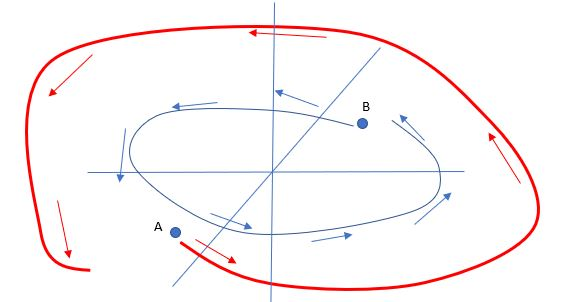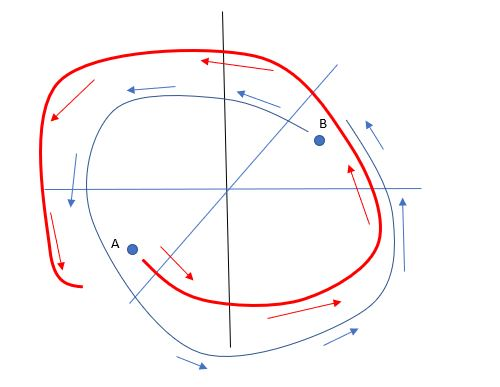# Coffee, anyon?Based on the stats I receive from WordPress.com, most readers of this blog live in the US, India and the UK. In addition, there are several readers in Canada, Saudi Arabia, China, Romania, Turkey, Nigeria and France. Suppose you live in the first three of these countries. In addition, let’s say you go to your favorite coffee shop (Starbucks, Cafe Coffee Day or your friendly neighbourhood Costa) and get a cup of coffee. Your coffee is brewed with hot water – usually filtered and quite pure, let’s even assume it is distilled, so it contains only$H_2 O$.

Now, here is an interesting question. Can you tell, from tasting the water, where it came from, which animals in the long history of the planet passed it through their digestive systems, or which supernova or neutron star collision chucked out the heavier elements that became these particular water molecules?

No, obviously, you can’t. Think of the chaos it would create – your coffee would taste different every day, for instance!

This is an interesting observation! It indicates that the way materials behave, they lose their memory of their past state. But isn’t this in apparent contradiction with the laws of classical mechanics that determine${\bf all}$ final conditions based on initial conditions. In fact, until the understanding of chaos and “mixing” came along, physicists and mathematicians spent a lot of time trying to reconcile this apparent contradiction.

When quantum mechanics came along, it allowed physicists to make sense of this in a rather simple way. Quantum states are superpositions of several microstates. In addition, when a measurement is made on a quantum system, it falls into one of the several microstates. Once it does, it has no memory of its previous superposition of states. However, there is yet another reason why history may not matter. This reason is only relevant in two spatial dimensions.

Let’s study some topology.

Suppose you have two particles, say two electrons. A quantum-mechanical system is described by a wave-function$\psi$, which is a function of the coordinates of the two particles, i.e.,$\psi(\vec x_1, \vec x_2)$. If the particles are${\bf {identical}}$, then one can’t tell which particle is the “first” and which the “second” – they are like identical twins and physically we cannot tell them apart from each other. So, the wave function$\psi$ might mean by its first argument, the first particle, or the second particle. How should the wave-function with one assumed order of particles be related to the wave-function with the “exchanged” order of particles? Are they the same wave function?

In three or more dimensions, the argument that’s made is as follows. If you exchange the two particles, then exchange them back again, you recover the original situation. So you should be back to your original wave function, correct? Not necessarily – the wave function is  a complex number. It is not exactly the quantity with physical relevance, only the square of the magnitude (which yields the probability of the configuration) is. So, the wave function could get multiplied by a complex phase – a number like$e^{i \theta}$ which has magnitude 1 and still be a perfectly good description of the same situation.

So if we had the particles A & B like this and switch themthe wave function could get multiplied by a phase$e^{i \theta}$ with the wave function describing an identical situation. But if we did the same operation and switched them again, we have to come back to exactly the same wave function as before –  we might need to multiply by the square of the above complex number$(e^{i \theta})^2$; however, this square has to be equal to$1$. Why in this case? Well, remember, it looks like we are back to the identical original situation and it would be rather strange if the same situation were described by a different wave function just because some one might or might not have carried out a double “switcheroo”.

If the square of some number is$1$, that number is either$1$ or$-1$. Those two cases correspond to either bosons or fermions – yes the same guys we met in a previous post. Now, why the exchange property determines the statistical properties is a subtle theorem called the spin-statistics theorem and it will be discussed in a future post.

However, in two dimensions, it is very obvious that something very peculiar can happen.

If you have switched two particles in three dimensions, look at the picture below.then let’s mould the switching paths for the total double switch as belowwe can move the “switcheroo” paths around and shrink each to a point. Do the experiment with  strings to convince yourself.

So, exchanging the two particles twice is equivalent to doing nothing at all.

However, if we were denizens of “Flatland” and lived on a two-dimensional flat space, we${\bf {cannot}}$ shrink those spiral shaped paths to a point – there isn’t an extra dimension to unwind the two loops and shrink them to a point. We cannot pull one of the strings out into the third dimension and unravel it from the other! So, there is no reason for two switches to bring you back to the original wave function for the two identical particles.${\bf {The \: \: particles \: \: remember \: \: where \: \: they \: \: came \: \: from}}$.This is why the quantum physics of particles in two dimensions is complicated – you can’t make the usual approximations to neglect the past and start a system off from scratch, the past be damned.

Particles in two dimensions are called anyons. Coffee with anyons in two dimensions would taste mighty funny and you would smell every damned place in two dimensions it had been in before it entered your two-dimensional mouth!